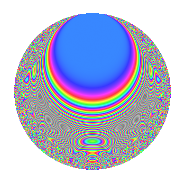# Properties

 Label 403.2.hLevel 403 Weight 2 Character orbit h Rep. character $$\chi_{403}(118,\cdot)$$ Character field $$\Q(\zeta_{3})$$ Dimension 64 Newform subspaces 2 Sturm bound 74 Trace bound 1

# Related objects

## Defining parameters

 Level: $$N$$ $$=$$ $$403 = 13 \cdot 31$$ Weight: $$k$$ $$=$$ $$2$$ Character orbit: $$[\chi]$$ $$=$$ 403.h (of order $$3$$ and degree $$2$$) Character conductor: $$\operatorname{cond}(\chi)$$ $$=$$ $$31$$ Character field: $$\Q(\zeta_{3})$$ Newform subspaces: $$2$$ Sturm bound: $$74$$ Trace bound: $$1$$ Distinguishing $$T_p$$: $$2$$

## Dimensions

The following table gives the dimensions of various subspaces of $$M_{2}(403, [\chi])$$.

Total New Old
Modular forms 80 64 16
Cusp forms 72 64 8
Eisenstein series 8 0 8

## Trace form

 $$64q - 4q^{3} + 56q^{4} + 2q^{5} + 4q^{7} + 12q^{8} - 36q^{9} + O(q^{10})$$ $$64q - 4q^{3} + 56q^{4} + 2q^{5} + 4q^{7} + 12q^{8} - 36q^{9} - 10q^{10} - 8q^{11} - 16q^{12} - 2q^{13} - 4q^{14} + 12q^{15} + 40q^{16} - 4q^{17} + 8q^{19} + 2q^{20} - 2q^{21} + 14q^{22} - 20q^{23} - 12q^{24} - 46q^{25} - 6q^{26} + 32q^{27} + 6q^{28} + 8q^{29} - 32q^{30} + 10q^{31} - 16q^{32} - 12q^{33} - 36q^{34} + 20q^{35} - 12q^{36} - 10q^{37} - 2q^{38} - 46q^{40} + 2q^{41} + 52q^{42} + 14q^{43} - 92q^{44} + 28q^{45} - 24q^{46} - 8q^{47} - 18q^{48} - 26q^{49} + 2q^{50} - 10q^{51} - 6q^{52} + 34q^{53} + 32q^{54} + 34q^{55} - 48q^{56} + 8q^{57} - 12q^{58} + 18q^{59} + 40q^{60} - 20q^{61} - 42q^{62} - 24q^{63} + 116q^{64} - 12q^{65} + 200q^{66} - 2q^{67} + 2q^{68} - 18q^{69} - 56q^{70} + 30q^{71} - 18q^{72} - 20q^{73} - 14q^{74} - 20q^{75} + 8q^{76} + 4q^{77} - 14q^{79} - 22q^{80} - 40q^{81} - 48q^{82} - 10q^{83} - 10q^{84} + 76q^{85} - 34q^{86} + 22q^{88} + 32q^{89} + 42q^{90} + 16q^{91} - 92q^{92} - 4q^{93} - 84q^{94} - 128q^{96} - 52q^{97} + 12q^{98} - 60q^{99} + O(q^{100})$$

## Decomposition of $$S_{2}^{\mathrm{new}}(403, [\chi])$$ into newform subspaces

Label Dim. $$A$$ Field CM Traces $q$-expansion
$$a_2$$ $$a_3$$ $$a_5$$ $$a_7$$
403.2.h.a $$30$$ $$3.218$$ None $$-6$$ $$-2$$ $$7$$ $$6$$
403.2.h.b $$34$$ $$3.218$$ None $$6$$ $$-2$$ $$-5$$ $$-2$$

## Decomposition of $$S_{2}^{\mathrm{old}}(403, [\chi])$$ into lower level spaces

$$S_{2}^{\mathrm{old}}(403, [\chi]) \cong$$ $$S_{2}^{\mathrm{new}}(31, [\chi])$$$$^{\oplus 2}$$

## Hecke characteristic polynomials

There are no characteristic polynomials of Hecke operators in the database